Next: Method for Occlusions Breaking Up: Occlusion Recovery Previous: Occlusion Recovery

## Method for Occlusions Preserving Surfaces and Occlusions Breaking Surfaces , .

Identifying Allowed Reconstructions
We rely on distance transforms . This method finds perimeter points that are actually facing the connecting area. Then we draw lines between the pixels facing each other with the Bresenham algorithm, as in . The method relies on distance transforms, which encode the distance (in pixels) to the nearest region point. Figure 7 (center) shows the distance transform of one of the matching areas in figure 7 (left). Figure 7 (right) shows the potentially reconstructible area between the two surfaces shown in figure 7 (left). The distance transforms records the distance to the nearest boundary point allowing an easy computation of the hypothesised occluded surface.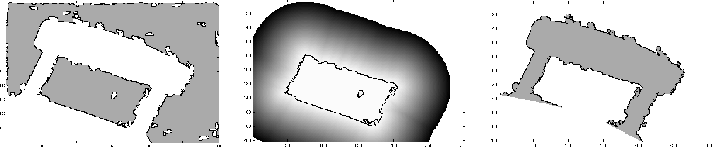Figure 7: Matching areas. These belong to the same wall lying behind the chair. Areas are gray, perimeter points are shown in black (left). The distance transform for one of the areas shown in the left figure. Perimeter points have been added for clarity. (right) The potentially reconstructible area between the two surfaces shown in the left figure.

Surface Reconstruction
Reconstruction takes place between regions belonging to the same group and satisfying the depth constraint. Given an occluding pixel and an occluded surface, a simple and intuitive way to perform reconstruction is to intersect the ray from the sensor through the occluding point with the occluded surface. As this ray overlaps the optical ray of the laser scanning beam, the reconstructed pixel is placed in a position that could actually have been sensed by the sensor.

For planes there is usually one intersection. For cylinders and spheres we usually find two intersections. If the shape of the surface is concave, the most distant intersection is chosen. Similarly on convex surfaces the closer intersection is chosen.

Due to noise, surfaces extrapolated into the possibly occluded area never perfectly match each other. Consequently, the surfaces are interpolated. A solution is a weighted averaging between all the intersections with the extrapolated surfaces, with weights depending on the distances from the intersection to the closest perimeter point of each surface. The position of the reconstructed pixel can be computed by: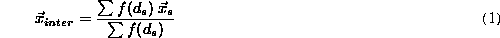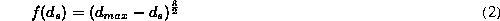The summation in equation (1) takes place over all the surfaces involved in the reconstruction. Because points that are closer to a certain surface would be weighted more than points that are further away, the weighting function should be decreasing with the input distance. The weighting function used for the example is shown in equation (2).

Reconstruction Validation
The choice now becomes whether to reconstruct or not. Reconstruction is a severe change to the image, so it is important to be very careful in applying it. If the area between two matching surfaces is further away from the sensor than the area of the surface to be reconstructed, then reconstruction should not happen (see figure 8). This happens on many occasions when niches as door or windows are involved. In cases of Occlusions Preserving Surfaces and Occlusions Breaking Surfaces, a proposed solution is to first reconstruct the surface and then compare reconstructed and measured points. Reconstruction is allowed only if reconstructed points lie behind the measured ones. All the pixels in the area between the surfaces are considered. To achieve a more reliable result each pixel vote for reconstruction, and only if enough pixels votes for a reconstruction will it be applied. As any reconstruction requires hypothesising non-observable data, the assumptions lead to a conservative reconstruction having a high likelihood of being correct.Figure 8: A real occlusion and a niche

Figure 4.1 shows some reconstructed images. The left image shows the occlusion caused by a chair in front of a wall. The right image is an image acquired by an orthographic scanner. The middle row shows a range image with reconstructed pixels and the bottom row shows the reconstruction in 3D. Because the reconstructed surface appeared unnaturally smooth, the same amount of Gaussian noise in the z-direction as found in the original surfaces was added.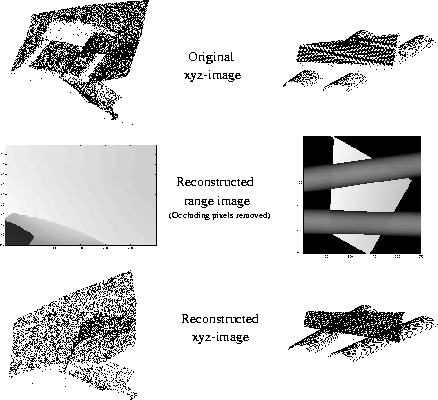Figure 9: Results for an occluded wall (left) and two occluded cylinders (right)

Texture Reconstruction
Although reconstructing the occluded surfaces makes the 3D models more realistic (no more chair-shaped holes as in the figure), they still lack intensity texture. The reconstructed surfaces are merely points in 3D space without any information about the light intensity or colour at that point. So, we hypothesise what the intensity on the surface would have been if it was possible to directly sense the surface.

The main idea is illustrated in diagram of figure 10 The reconstructed points and the neighbouring measured ones are mapped onto a planar texture space. This step allows easier and more efficient processing than the unordered points in 3D space. For 3D scene planes, this is a simple translation and rotation. For cylinders we use a h-surface parametrization, and for spheres a-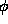parametrization.

An acquired intensity image is mapped on the same plane according to number and position of the mapped surface points and then propogated by a variety of methods. The reconstructed 3D points then acquire a hypothesized intensity from the corresponding point in the surface texture space.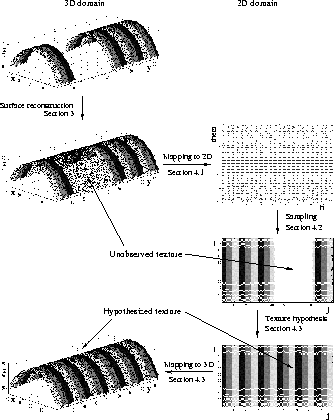Figure 10: Texture reconstructionNext: Method for Occlusions Breaking Up: Occlusion Recovery Previous: Occlusion Recovery

Bob Fisher
Thu Jan 17 16:45:14 GMT 2002